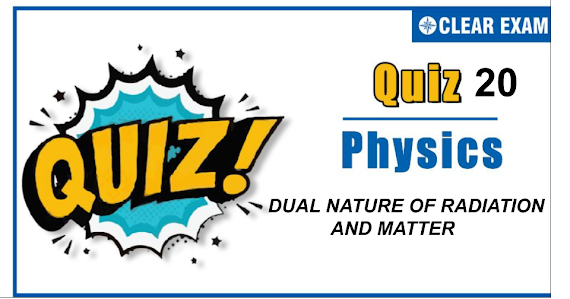## DUAL NATURE OF RADIATION AND MATTER quiz-20

As per analysis for previous years, it has been observed that students preparing for NEET find Physics out of all the sections to be complex to handle and the majority of them are not able to comprehend the reason behind it. This problem arises especially because these aspirants appearing for the examination are more inclined to have a keen interest in Biology due to their medical background. Furthermore, sections such as Physics are dominantly based on theories, laws, numerical in comparison to a section of Biology which is more of fact-based, life sciences, and includes substantial explanations. By using the table given below, you easily and directly access to the topics and respective links of MCQs. Moreover, to make learning smooth and efficient, all the questions come with their supportive solutions to make utilization of time even more productive. Students will be covered for all their studies as the topics are available from basics to even the most advanced. .

Q1. Ultraviolet light of wavelength 300 nm and intensity 1.0 Wm-2 falls on the surface on photoelectric metal. If one percent of incident photons produce photoelectrons, then the number of photoelectrons emitted from an area of 1.0 cm2 of the surface is nearly
•  2.13×1011 s-1
•  1.5×1012 s-1
•  3.02×1012 s-1
•  None of these
Solution
Energy of each photon =hc/λ = (6.6×10-34×3×108)/(300×10-9) = 6.6×10-19 J Power of source is given by p= intensity × area = 1.0×1.0×10-4 W = 10-4 W No. of photons per second = p/e=10-4/(6.6×10-19) Now number of electron emitted = 1/100×(10-4 /6.6×10-19 ) =1.5×1012 s-1

Q2.Which of the following statement about photon is incorrect?
•   Photons exert no pressure
•   Momentum of photon is hv/c
•   Photon’s rest mass is zero
•   Photon’s energy is hv
Solution
Photos move with velocity of light and have energy hv. Therefore, they also exert pressure

Q3. The wavelength of the matter wave is independent of
•  Mass
•  Velocity
•  Momentum
•  Charge
Solution
Charge

Q4. For moving ball of cricket, the correct statement about de-Broglie wavelength is
•  It is not applicable for such big particle
•  h/√2mE
•  √(h/2mE)
•  h/2mE
Solution
h/√2mE

Q5.In above question the energy of the characteristic X-rays given out is
•  Less than 40 keV
•  More than 40 keV
•  Equal to 40 keV
•  ≥40 keV
Solution
Less than 40 keV

Q6. Light of frequency v is incident on a substance of threshold frequency v0 (v0<v). The energy of the emitted photoelectron will be
•  h(v-v0)
•  h/v
•  he(v-v0)
•  h/v0
Solution
h(v-v0)

Q7.Cathode rays are similar to visible light rays as
•  They both can be deflected by electric and magnetic fields
•  They both have a definite magnitude of wavelength
•  They both can ionize a gas through which they pass
•  They both can expose a photographic plate
Solution
Light consists of photons and cathode rays consists of electrons. However both effect the photographic plate

Q8.A photo cell is receiving light from a source placed at a distance of 1 m. If the same source is to be placed at a distance of 2 m, then the ejected electron
•  Moves with one-fourth energy as that of the initial energy
•  Moves with one-fourth of momentum as that of the initial momentum
•  Will be half in number
•  Will be one-fourth in number
Solution
Number of ejected electrons ∝ (Intensity) ∝1/(Distance)2 Therefore an increment of distance two times will reduce the number of ejected electrons to 1/4 th of the initial value

Q9.According to Mosely’s law, the frequency of a spectral line in X-ray spectrum varies as
•  Atomic number of the element
•  Square of the atomic number of the element
•  Square ropot of the atomic number of the element
•  Fourth power of the atomic number of the element
Solution
Square of the atomic number of the element

Q10. Electron with energy 80 keV are incident on the tungsten target of a X-rays tube. Kshell electrons of tungsten have -72.5keV energy. X-rays emitted by the tube contain only
•  A continuous X-rays spectrum (Bremsstrahlung) with a minimum wavelength of ~0.155Å
•  A continuous X-ray spectrum (Bremsstrahlung) with all wavelengths
•  The characteristic X-rays spectrum of tungsten
• A continuous X-rays spectrum (Bremsstrahlung) with a minimum wavelength of ~0.155 Å and the characteristic X-rays spectrum of tungsten
Solution
Since the energy of incident electron, E=80 keV. The minimum wavelength of X-rays produced is λ=hc/E=(6.6×10-34×3×108)/(80×1000×1.6×10-19 ) =1.55×10-10 m=0.155 Å Since the energy of K-shell electron is -72.5 keV, so the incident electron of energy 80 keV will not only produce continuous spectrum of minimum wavelength 0.155Å but shell also knock electron of K shell out of atom, resulting emission of characteristics X-rays## Want to know more

Please fill in the details below:

## Latest NEET Articles\$type=three\$c=3\$author=hide\$comment=hide\$rm=hide\$date=hide\$snippet=hide

Name

ltr
item
BEST NEET COACHING CENTER | BEST IIT JEE COACHING INSTITUTE | BEST NEET, IIT JEE COACHING INSTITUTE: QUIZ-20 DUAL NATURE OF RADIATION AND MATTER
QUIZ-20 DUAL NATURE OF RADIATION AND MATTER